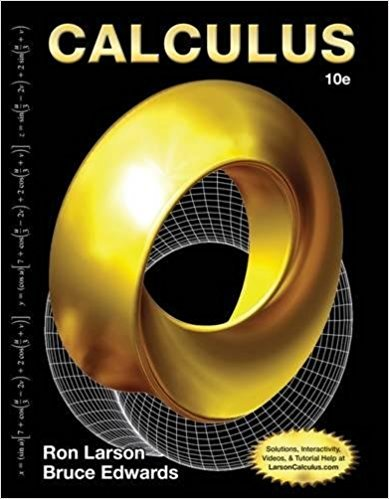×
×

# Solutions for Chapter 9: Calculus 10th Edition## Full solutions for Calculus | 10th Edition

ISBN: 9781285057095Solutions for Chapter 9

Solutions for Chapter 9
4 5 0 403 Reviews
25
1
##### ISBN: 9781285057095

Chapter 9 includes 16 full step-by-step solutions. This textbook survival guide was created for the textbook: Calculus, edition: 10. This expansive textbook survival guide covers the following chapters and their solutions. Since 16 problems in chapter 9 have been answered, more than 2689 students have viewed full step-by-step solutions from this chapter. Calculus was written by and is associated to the ISBN: 9781285057095.

Key Calculus Terms and definitions covered in this textbook
• Acute angle

An angle whose measure is between 0° and 90°

• DMS measure

The measure of an angle in degrees, minutes, and seconds

• Ellipse

The set of all points in the plane such that the sum of the distances from a pair of fixed points (the foci) is a constant

• Inverse reflection principle

If the graph of a relation is reflected across the line y = x , the graph of the inverse relation results.

• Limaçon

A graph of a polar equation r = a b sin u or r = a b cos u with a > 0 b > 0

• Linear regression equation

Equation of a linear regression line

• Magnitude of a vector

The magnitude of <a, b> is 2a2 + b2. The magnitude of <a, b, c> is 2a2 + b2 + c2

• Modulus

See Absolute value of a complex number.

• Obtuse triangle

A triangle in which one angle is greater than 90°.

• Power rule of logarithms

logb Rc = c logb R, R 7 0.

• Product of complex numbers

(a + bi)(c + di) = (ac - bd) + (ad + bc)i

• Pseudo-random numbers

Computer-generated numbers that can be used to approximate true randomness in scientific studies. Since they depend on iterative computer algorithms, they are not truly random

• Rational function

Function of the form ƒ(x)/g(x) where ƒ(x) and g(x) are polynomials and g(x) is not the zero polynomial.

• Real part of a complex number

See Complex number.

• Relation

A set of ordered pairs of real numbers.

• Solve by substitution

Method for solving systems of linear equations.

• System

A set of equations or inequalities.

• Third quartile

See Quartile.

• Transitive property

If a = b and b = c , then a = c. Similar properties hold for the inequality symbols <, >, ?, ?.

• Unit ratio

See Conversion factor.

×